Home / Circuits / How to Use the LM2596S Module

# How to Use the LM2596S Module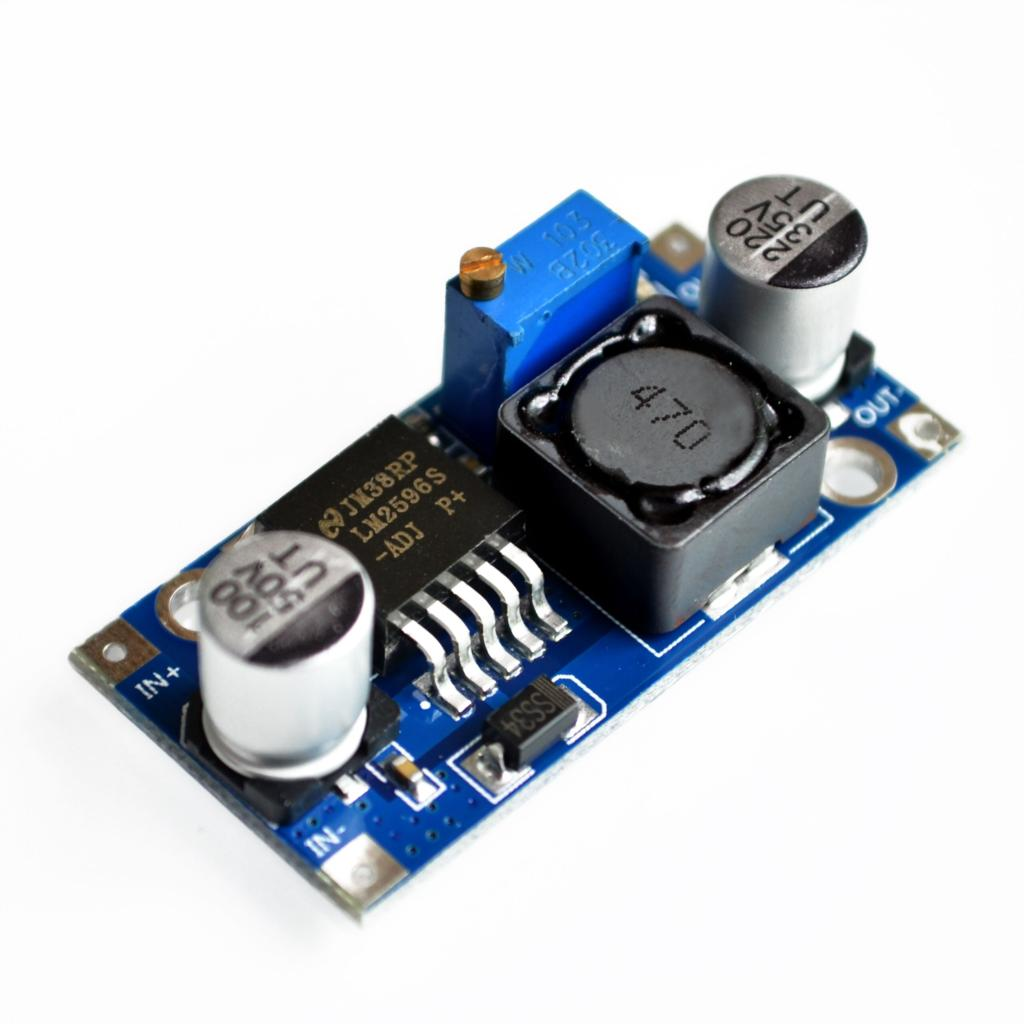The LM2596S module is an easy to use voltage regulator for people who use multiple power sources in their projects.

### Introduction to LM2596S

The module features the LM2596S SIMPLE SWITCHER® Power Converter from National Instruments. This voltage regulator IC can provide fixed voltages of 3.3, 5 and 12 V as well as a variable output. Moreover, the LM2596S safely belts out a maximum of 3 A current to its load.

The LM2596S requires only 4 external components to work. Below is an application circuit for fixed output voltage: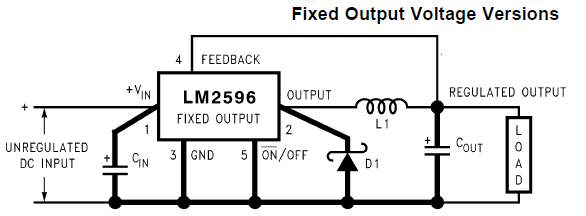As seen above, the designer only needs to determine the values of CIN, COUT, L1 and D1 base on his/her requirements.

For example, this circuit provides a regulated 5 VDC for an unregulated 12 VDC output: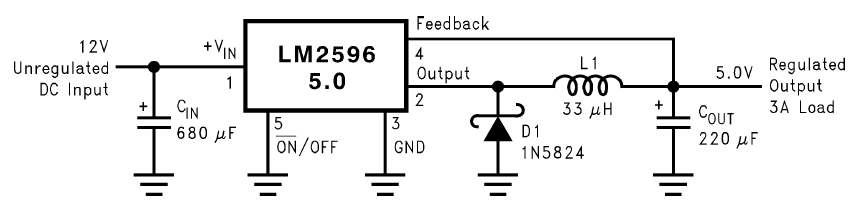Meanwhile this circuit provides an adjustable voltage output: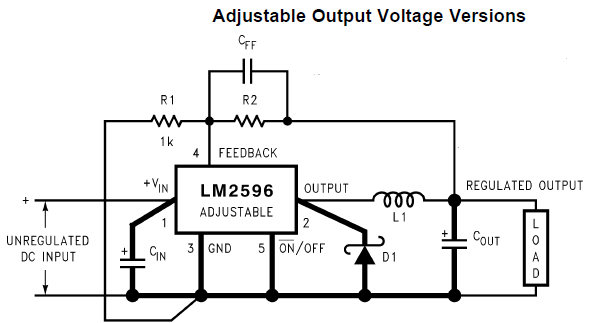The output voltage is calculated using the formula: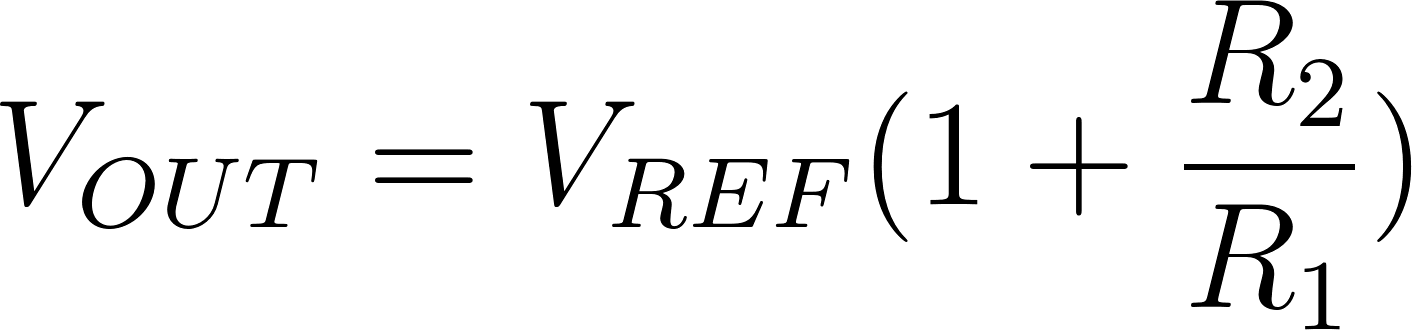### LM2596S Module

The easiest way to use the LM2596S, obviously, is to use the module. The module shown below has two input pins and two output pins:You only need to connect an input voltage to the IN pins (taking note of the correct polarity) and then a regulated DC voltage will be on the OUT pins. The input voltage must be higher than the output voltage at least 1.5 V. For example, if you need an output of 7 V, the input must be at least 8.5 V.

To adjust the output, carefully turn the potentiometer knob using a non-metallic screwdriver.

Below an example circuit where a 12 VDC source is used to power both a microcontroller and a solenoid: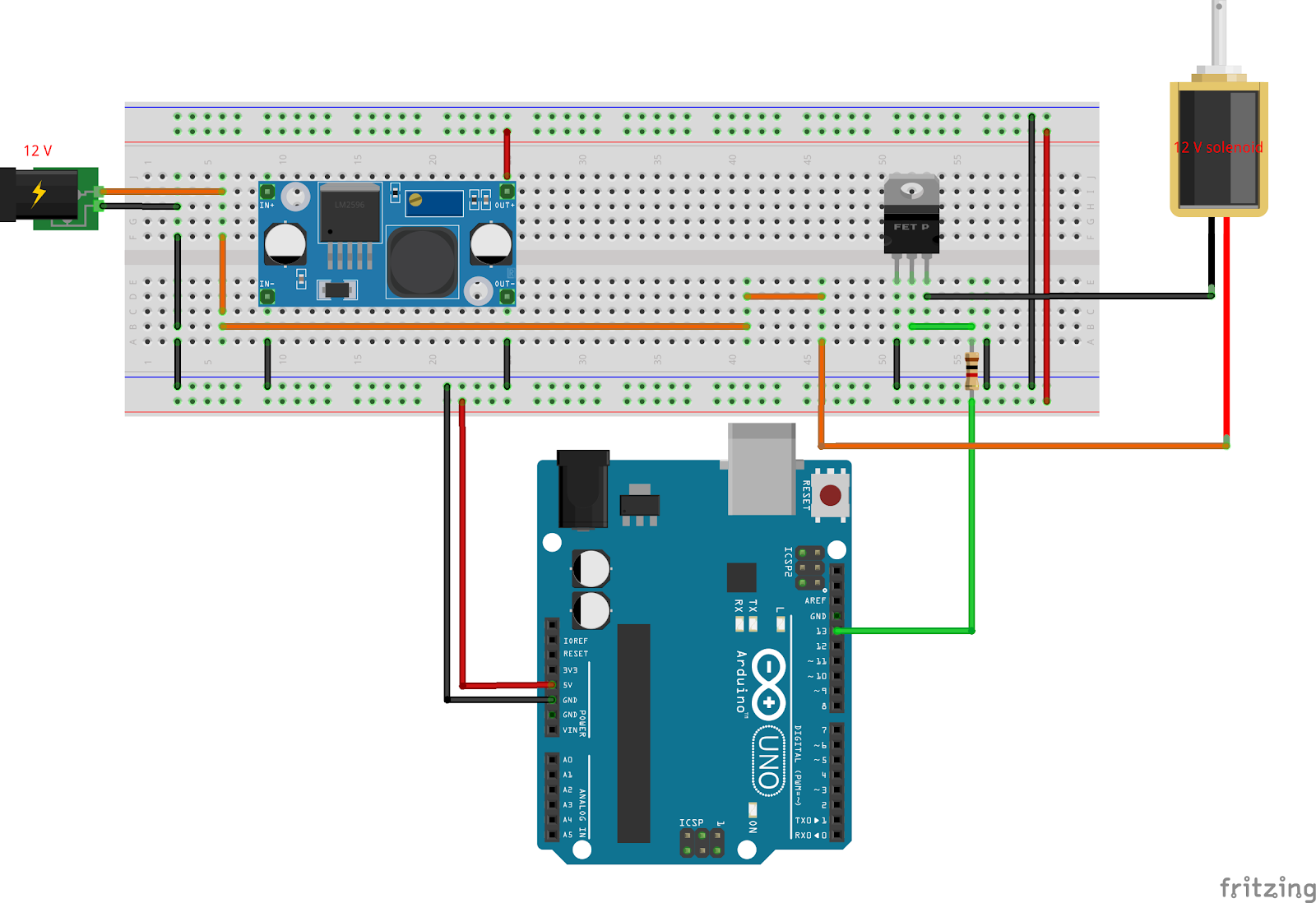Here, the 12 V solenoid is triggered by a 5 V arduino with a FET acting as the switch. The 12 V source needed to drive the solenoid is isolated from the Arduino, preventing the latter from being damaged.

## Voltage Divider Calculator

Introduction to Voltage Dividers Resistors are in series when the same current flows through them. …